# Hardest colleges to get into in Pennsylvania

Top 10 colleges in Pennsylvania with the lowest acceptance rates
What are the hardest colleges to get into in Pennsylvania? We've got you covered. We've compiled a national college database and have created a list of the hardest universities to get into in Pennsylvania below. These are the hardest 4 year colleges to get into in Pennsylvania, and sometimes some of the hardest colleges to get into in the US. You could even say these are the best colleges in Pennsylvania. We also include each college's average SAT and ACT scores so that you can see where you're most competitive. Read on to find out more.

## University of Pennsylvania acceptance rate

University of Pennsylvania acceptance rate is 8.4%.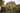The average SAT score for University of Pennsylvania is 1500.

• SAT composite: 1500
• SAT math: 770

The average ACT score for University of Pennsylvania is 34.

## Swarthmore College acceptance rate

Swarthmore College acceptance rate is 9.5%.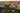The average SAT score for Swarthmore College is 1470.

• SAT composite: 1470
• SAT math: 750

The average ACT score for Swarthmore College is 32.

## Carnegie Mellon University acceptance rate

Carnegie Mellon University acceptance rate is 15.4%.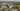The average SAT score for Carnegie Mellon University is 1510.

• SAT composite: 1510
• SAT math: 780

The average ACT score for Carnegie Mellon University is 34.

## Haverford College acceptance rate

Haverford College acceptance rate is 18.8%.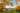The average SAT score for Haverford College is 1450.

• SAT composite: 1450
• SAT math: 740

The average ACT score for Haverford College is 33.

## Villanova University acceptance rate

Villanova University acceptance rate is 28.2%.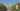The average SAT score for Villanova University is 1395.

• SAT composite: 1395
• SAT math: 715

The average ACT score for Villanova University is 33.

## Lafayette College acceptance rate

Lafayette College acceptance rate is 31.5%.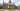The average SAT score for Lafayette College is 1325.

• SAT composite: 1325
• SAT math: 665

The average ACT score for Lafayette College is 31.

## Lehigh University acceptance rate

Lehigh University acceptance rate is 32.1%.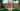The average SAT score for Lehigh University is 1365.

• SAT composite: 1365
• SAT math: 710

The average ACT score for Lehigh University is 31.

## Bucknell University acceptance rate

Bucknell University acceptance rate is 33%.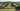The average SAT score for Bucknell University is 1337.

• SAT composite: 1337
• SAT math: 680

The average ACT score for Bucknell University is 30.

## Bryn Mawr College acceptance rate

Bryn Mawr College acceptance rate is 34.1%.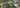The average SAT score for Bryn Mawr College is 1410.

• SAT composite: 1410
• SAT math: 710

The average ACT score for Bryn Mawr College is 31.

## Franklin & Marshall College acceptance rate

Franklin & Marshall College acceptance rate is 35.3%.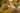The average SAT score for Franklin & Marshall College is 1360.

• SAT composite: 1360
• SAT math: 700